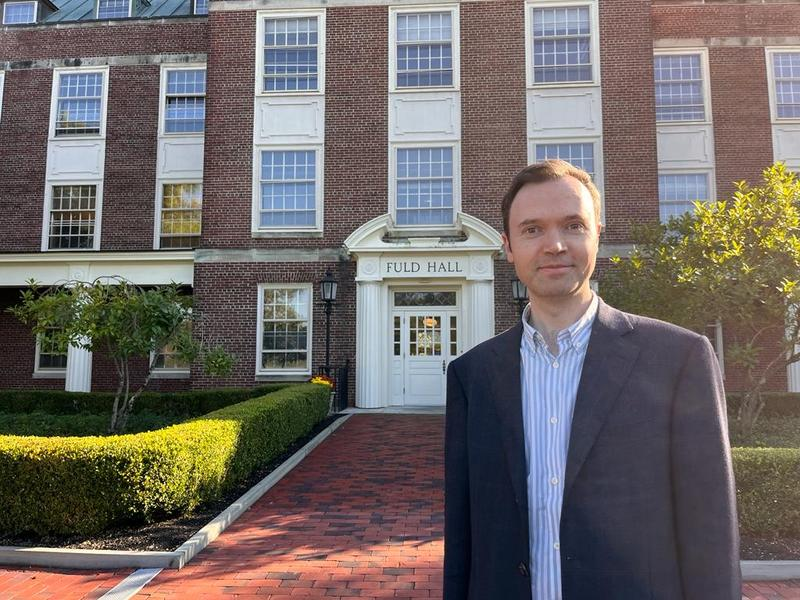# Thomas CassThomas Cass is a Reader at the Mathematics Department at Imperial College London. He obtained his MA and PhD from Department of Pure Mathematics and Mathematical Statistics at the University of Cambridge.

Thomas's research interests relate to the study of random phenomena. His research writings span both classical areas of stochastic analysis such as Malliavin calculus and Gaussian processes as well as more recent aspects of rough path analysis. He is also interested in the way in which insights in pure mathematics can spur developments in mathematical finance and in data science.

A more specific list of his interests includes:

• the theory of rough paths, rough differential equations and rough analysis.
• Gaussian processes, fractional Brownian motion and the properties of rough differential equations driven by these processes
• the use of Malliavin calculus and cubature on Wiener space for sensitivity analysis and efficient pricing and hedging methods in mathematical finance
• McKean-Vlasov-type models for large populations of interacting particles and agents
• stochastic differential geometry and rough paths on manifolds
• the signature and its mathematics, and applications of rough paths to data science, especially in radioastronomy.